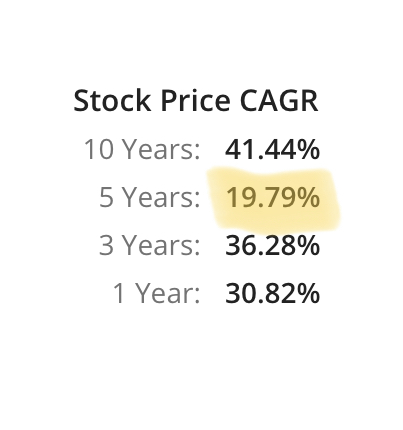# Stock Return Calculation

Hello. Can someone please help me in understanding the way to calculate the return while i am not able to differentiate between the different way of Return Calculations.

Here in the first screenshot where it’s mentioned that the 5 Return Of Relaxo is 146.6% (looks so Great) .

But in the second screenshot, where it is mentioned that 5year CAGR is just 19.79%. Which means my money grew every year with 19.79% for 5 year?

which return is to be taken to compare 2 stocks? CAGR?

And If we are selecting CAGR, then why in the website it is showing return as 146%.1 Like

How are you getting this figure?

CAGR calculator. I feeded the initial amount with the mentioned CAGR percent for 5 year in the calculator

The 5 year return is indeed 146.6% i.e. after a period of 5 years the stock had incread by that much. Now, 19.79 is the compounded return of that stock over 5 years. Its just another way to look at things. If you want calculations then -

Investment amount - Rs100 CAGR - 19.79%

CAGR means that 19.79% will be added to the principle each year but the principle would be considered the resultant capital of previous year. So-

After 1 year - 119.79 (100+19.79%)
After 2 years - 143.49 (119.79 + 19.79%)
After 3 years - 171.88 (143.49 + 19.79%)
After 4 years - 205.9 (171.88 + 19.79%)
After 5 years - 246.65 (205.9 + 19.79%)

So, profit earned = 246.65 - 100 = 146.65

Which if you calculate is 146.65% return. Difference of 0.01 percent is there because i ignored the decimals after first 2 places while calculating.

But you get the idea. It’s the same thing written in 2 different ways. You can take whichever you want.

4 Likes

To compare two investment which has different absolute return and holding period, why can’t we use annual absolute return (absolute return/holding period) to compare two investment rather than CAGR?